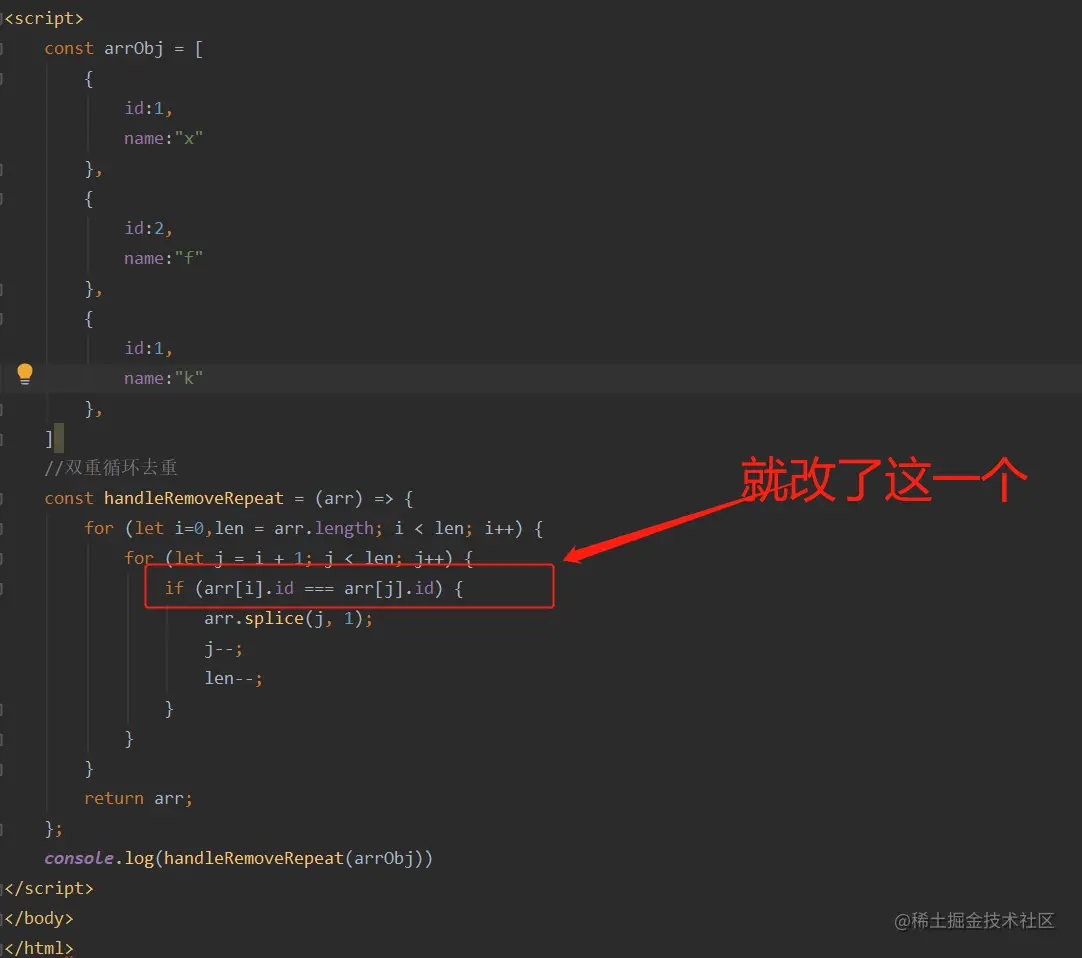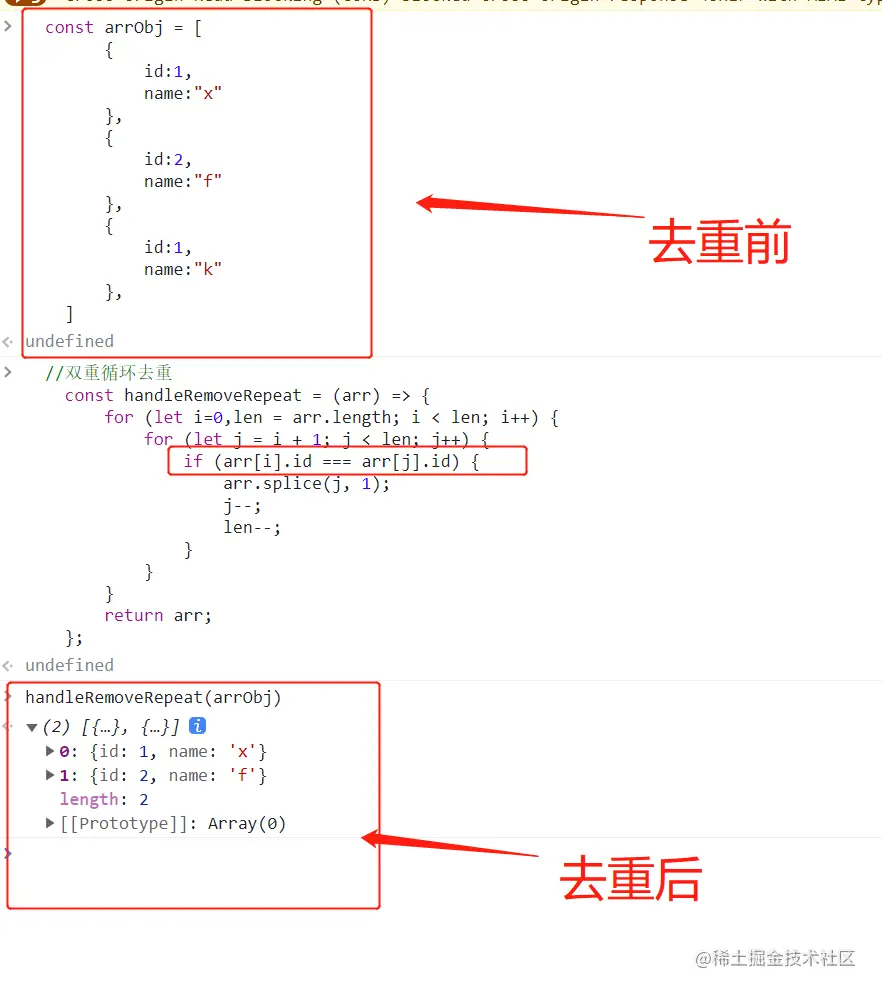# 【面试题】数组去重的五种方法（必会）# 五种方式

### 最简单的方法，ES6的Set去重(最推荐)

new Set是ES6新推出的一种类型。他和数组的区别在于，Set类型中的数据不可以有重复的值。当然，数组的一些方法Set也无法调用。

``````    const arr = [1,1,2,2,3,3,4,4,5,5];
const setData = Array.from(new Set(arr));
console.log(setData);
``````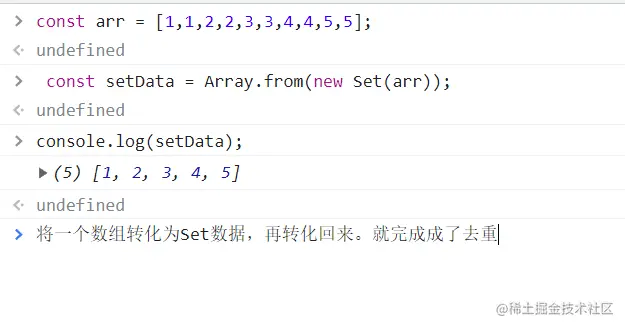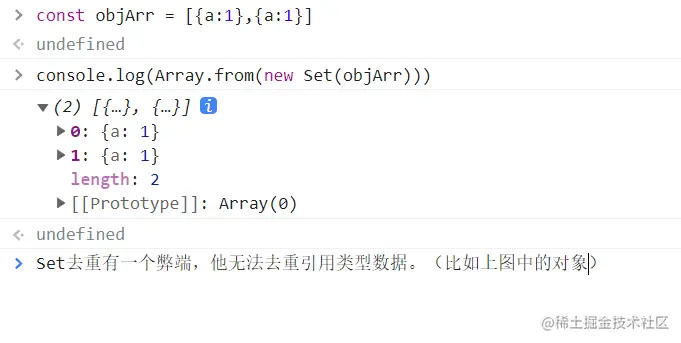### 最古老的方法，双重for循环去重

``````//双重循环去重
const handleRemoveRepeat = (arr) => {
for (let i=0,len = arr.length; i < len; i++) {
for (let j = i + 1; j < len; j++) {
if (arr[i] === arr[j]) {
arr.splice(j, 1);
j--;
len--;
}
}
}
return arr;
};
``````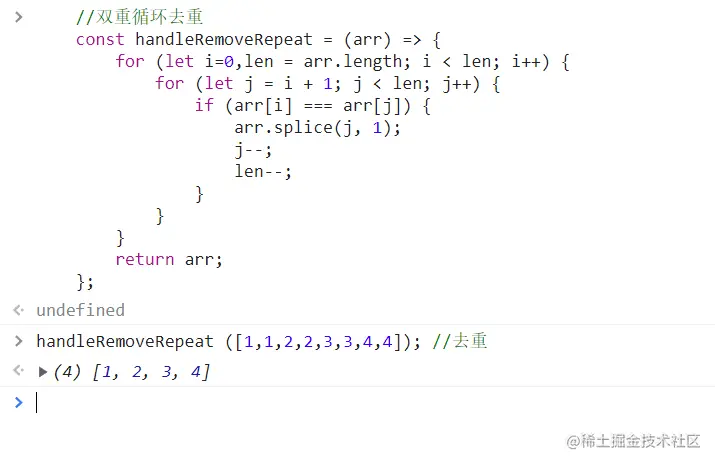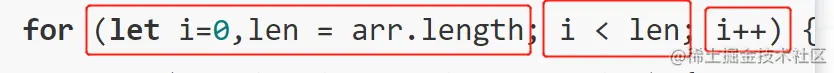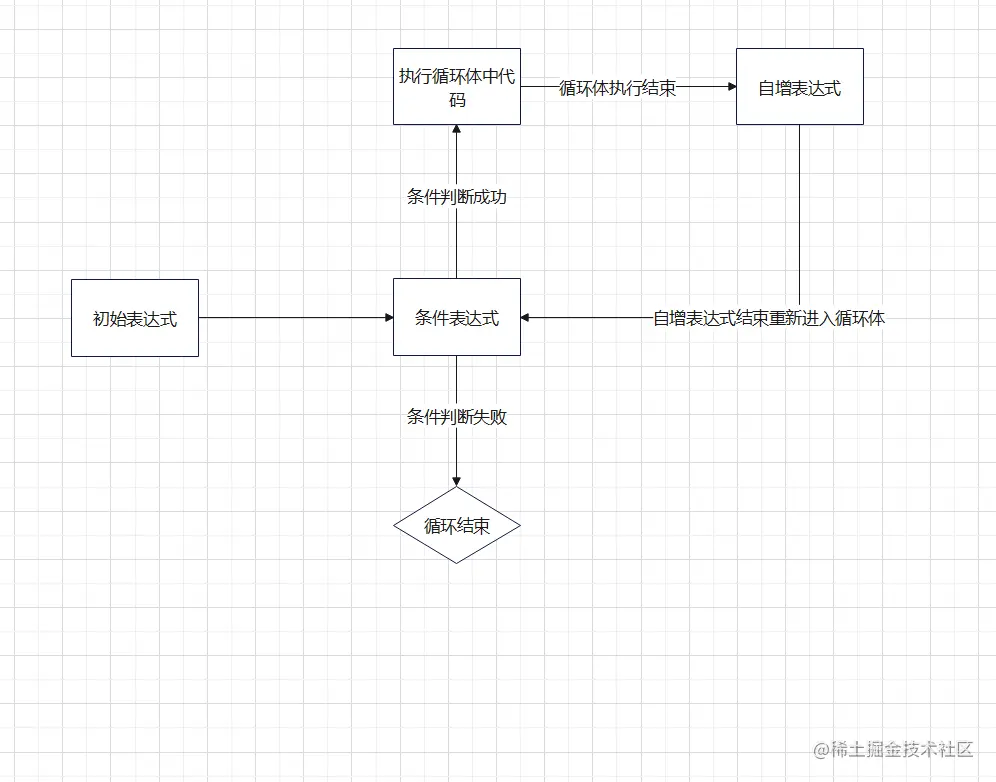### 最鸡肋的去重方式，indexOf去重

indexOf还是相对简单又鸡肋。为什么说他鸡肋呢?说难吧，indexOf方法确实比上文的双重for循环简单。说简单吧，嘿，他没Set方法去重来的更简单。所以鸡肋。

``````//去重
const handleRemoveRepeat = (arr) => {
let repeatArr = [];
for (let i = 0,len = arr.length ; i < len; i++)
if (repeatArr.indexOf(arr[i]) === -1)  repeatArr.push(arr[i])
return repeatArr;
}
``````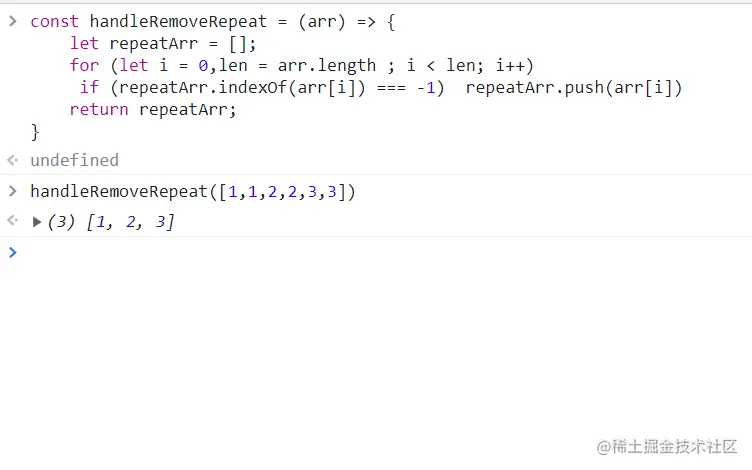### 一种类似于indexOf的去重方法，includes去重

includes的判断方法更简单了。循环数组的每一样，用新数组检测当前数组中是否包含数组项，如果不包含，则追加该元素

``````const handleRemoveRepeat = (arr) => {
let repeatArr = [];
for (let i = 0,len = arr.length ; i < len; i++)
if (!repeatArr.includes(arr[i])) repeatArr.push(arr[i])
return repeatArr;
}
``````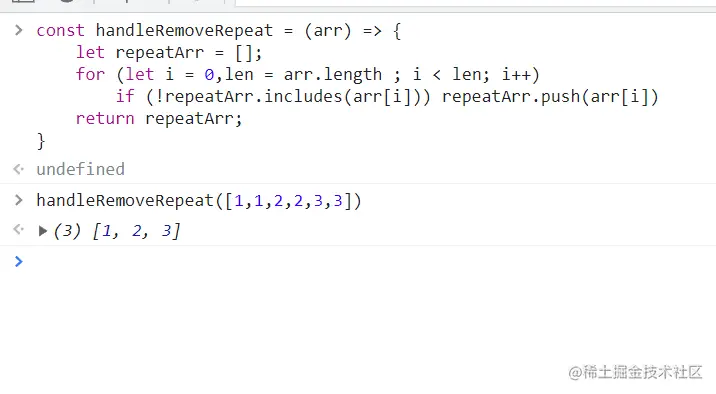includes方法在除了去重以外的场景，还是很好用的。

### 最有趣的去重方法，使用filter去重。

``````//去重
const handleRemoveRepeat = (arr) => arr.filter((item,index) => arr.indexOf(item,0) === index);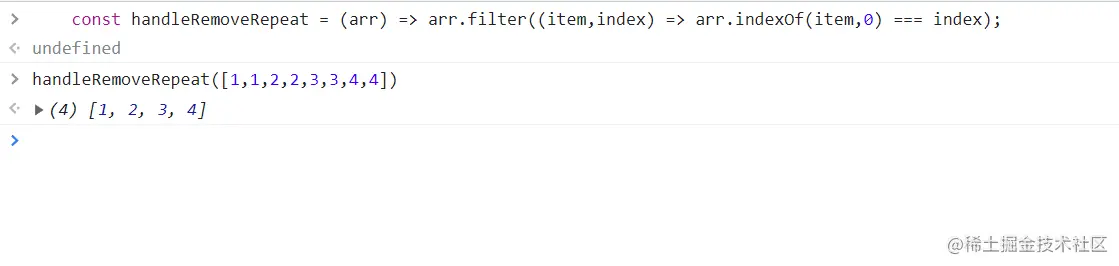indexOf的特性是返回被查找的目标中包含的第一个位置的索引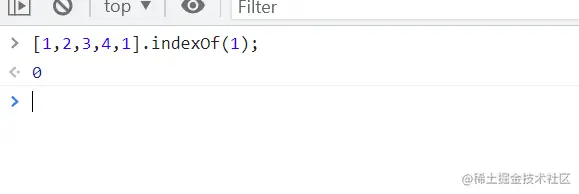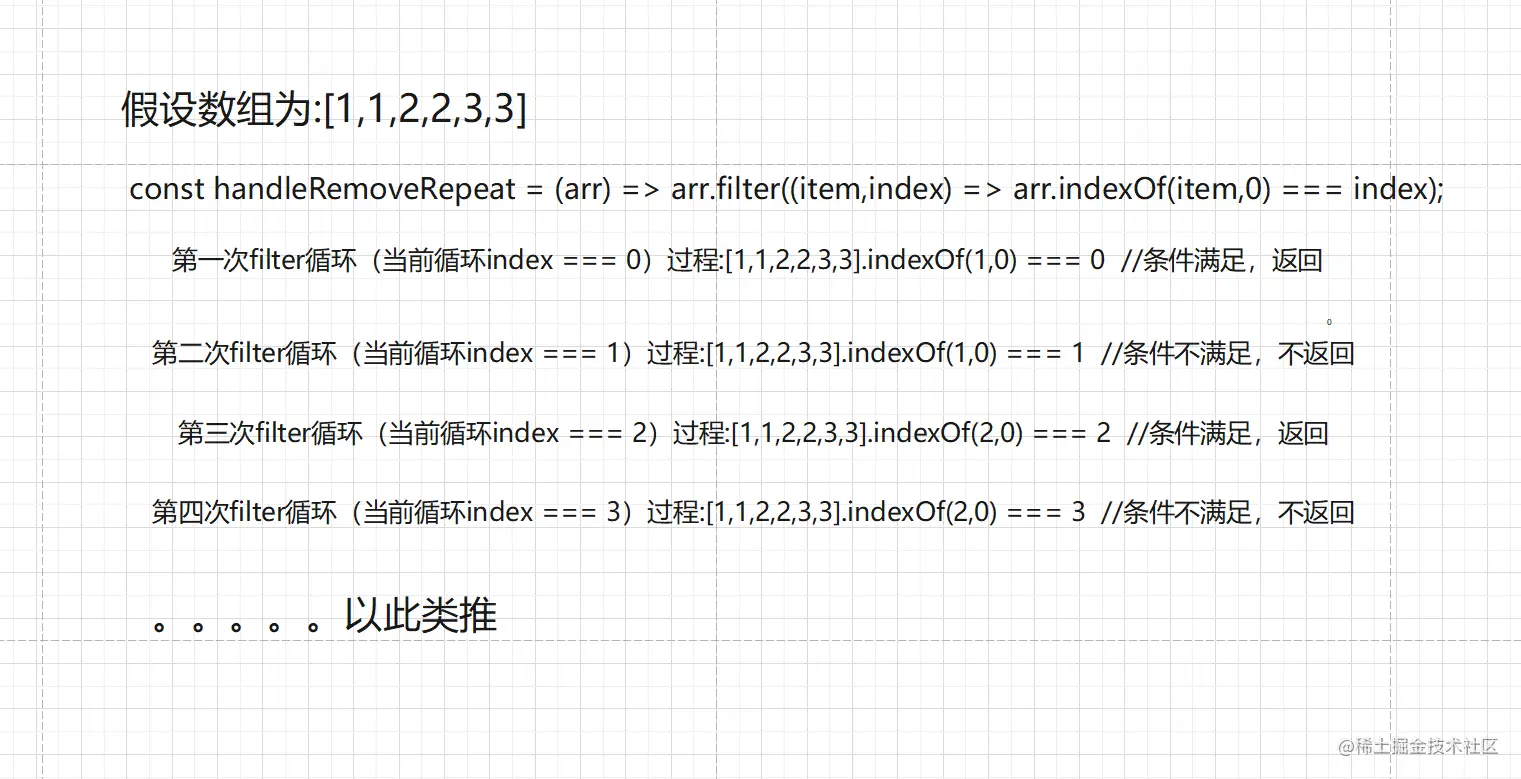### 您或许会问:如果要去重对象数组怎么办？